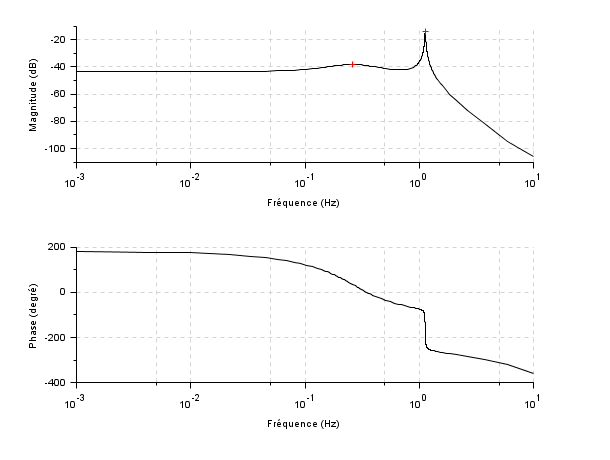Scilab Home page | Wiki | Bug tracker | Forge | Mailing list archives | ATOMS | File exchange
Change language to: Français - Português - 日本語 - Русский

See the recommended documentation of this function

# freson

peak frequencies

### Syntax

`fr=freson(h)`

### Arguments

h

A siso linear dynamical system, in state space, transfer function or zpk representations.

fr

vector of peak frequencies in Hz

### Description

returns the vector frequencies at which the response amplitude of a siso dynamical system is a relative maximum also called resonance frequencies.

### Examples

Continuous time system

```h=syslin('c',-1+%s,(3+2*%s+%s^2)*(50+0.1*%s+%s^2));
fr=freson(h)
clf;bode(h);
fig=gcf();sca(fig.children(2));
[phi,db]=phasemag(repfreq(h,fr));
plot(fr,db(:),'+r')```Discrete time system

```hd=dscr(h,0.01)
fr=freson(hd)
clf;bode(hd);
fig=gcf();sca(fig.children(2));
[phi,db]=phasemag(repfreq(hd,fr));
plot(fr,db(:),'+r');```• frep2tf — transfer function realization from frequency response
• zgrid — zgrid plot
• h_norm — H-infinity norm

### History

 Version Description 6.0 handling discrete systems and zpk representation, peak detection improved.